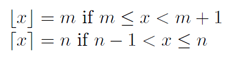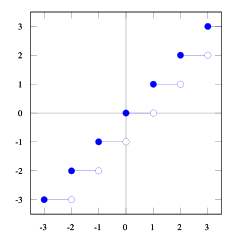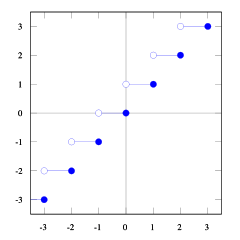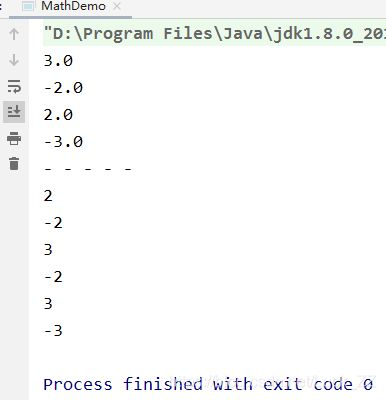• Math.round()，Math.ceil()，Math.floor()的区别
2021-04-09 16:07:54

1.Math.round()：根据“round”的字面意思“附近、周围”，可以理解该函数是求一个附近的整数，看下面几个例子就明白。

小数点后第一位<5

正数：Math.round(11.46)=11

负数：Math.round(-11.46)=-11

小数点后第一位>5

正数：Math.round(11.68)=12

负数：Math.round(-11.68)=-12

小数点后第一位=5

正数：Math.round(11.5)=12

负数：Math.round(-11.5)=-11

总结：（遵循四舍五入，小数点后第一位=5？负数最大取整，如，Math.round(-11.5)= -11        ）     （小数点后第一位）大于五全部加，等于五正数加，小于五全不加。

2.Math.ceil()：根据“ceil”的字面意思“天花板”去理解；

例如：

Math.ceil(11.46)=Math.ceil(11.68)=Math.ceil(11.5)=12

Math.ceil(-11.46)=Math.ceil(-11.68)=Math.ceil(-11.5)=-11

总结：（不管四舍五入）向上最大的取整。        PS：注意负数，是负数的最大整数，比如：Math.ceil(-11.68)= -11

3.Math.floor()：根据“floor”的字面意思“地板”去理解；

例如：

Math.floor(11.46)=Math.floor(11.68)=Math.floor(11.5)=11

Math.floor(-11.46)=Math.floor(-11.68)=Math.floor(-11.5)=-12

总结：（不管四舍五入）向下最小的取整。        PS：注意负数，是负数的最大整数，比如：Math.ceil(-11.68)= -11

更多相关内容
• Math.ceil(x) -- 返回大于等于数字参数的最小整数(取整函数)，对数字进行上舍入，下面有个不错的示例，感兴趣的朋友可以参考下
• 主要介绍了Java Math.round()，Math.ceil()，Math.floor()的区别详解，文中通过示例代码介绍的非常详细，对大家的学习或者工作具有一定的参考学习价值，需要的朋友们下面随着小编来一起学习学习吧
• Math.ceil(): round a number up Arguments: Any numeric value or expression Returns: The closest integer greater than or equal to x. ———————————————————————————————– Math...
• js Math.ceil用于对数值向上取整，即得到大于或等于该数值的最小整数,需要的朋友可以参考下
• int total = (int) Math.ceil(157/32);为什么仍然返回4？ 157/32 = 4.90625，我需要四舍五入，我环顾四周，这似乎是正确的方法。我尝试将total作为double类型，但得到4.0。我究竟做错了什么？您正在执行157/32，该...

int total = (int) Math.ceil(157/32);

为什么仍然返回4？ 157/32 = 4.90625，我需要四舍五入，我环顾四周，这似乎是正确的方法。

我尝试将total作为double类型，但得到4.0。

我究竟做错了什么？

您正在执行157/32，该操作将两个整数彼此相除，这总是导致四舍五入的整数。因此，(int) Math.ceil(...)没有执行任何操作。有三种可能的解决方案来实现您想要的。我建议使用选项1或选项2。请不要使用选项0。

选项0

将a和b转换为双精度，然后可以根据需要使用除法和Math.ceil。但是，我强烈不鼓励使用这种方法，因为双重除法可能不精确。要阅读有关双精度不精确性的更多信息，请参见此问题。

int n = (int) Math.ceil((double) a / b));

选项1

int n = a / b + ((a % b == 0) ? 0 : 1);

如果a和b都是整数，则始终将a / b设为最低。然后，您有一个内联的if语句女巫检查您是否应该天花板而不是地板。所以+1或+0，如果除法除法运算余数，则需要+1。 a % b == 0检查其余部分。

选项2

此选项很短，但可能不太直观。我认为这种不太直观的方法会比双重除法和比较方法更快：

int n = (a + b - 1) / b;

为了减少溢出的机会，您可以使用以下方法。但是请注意，它不适用于a = 0和b < 1。

int n = (a - 1) / b + 1;

"不太直观的方法"背后的解释

由于用Java(和大多数其他编程语言)将两个整数相除将始终使结果无效。所以：

int a, b;

int result = a/b (is the same as floor(a/b) )

但是我们不希望floor(a/b)，而是ceil(a/b)，并使用Wikipedia的定义和图解：使用floor和ceil函数的这些图，您可以看到关系。您可以看到floor(x) <= ceil(x)。我们需要floor(x + s) = ceil(x)。因此，我们需要找到s。如果我们采用1/2 <= s < 1，它将是正确的(尝试一些数字，您会看到的，我发现自己很难证明这一点)。还有1/2 <= (b-1) / b < 1

ceil(a/b) = floor(a/b + s)

= floor(a/b + (b-1)/b)

= floor( (a+b-1)/b) )

这不是真实的证明，但我希望您对此感到满意。如果有人可以更好地解释它，我也将不胜感激。也许在MathOverflow上询问。

如果您能解释不太直观的方法背后的直觉，将是一个极大的帮助？我知道这是正确的，我想知道您是如何做到的，以及如何从数学上证明它是正确的。我不敢相信，我试图用数学方法解决它。

希望您对我的编辑感到满意，我认为我不能做得更好:(

我假设Math.floor和ceil仅适用于整数除法，不适用于将值强制转换为双精度的长除法。反例是4611686018427386880/4611686018427387137在地板上失败和4611686018427386881/4611686018427386880在天花板上失败

我的计算机为Math.floor( (double) a (double) b)打印0.0，其中long a = 4611686018427386880L; long b = 4611686018427387137L;，所以我想我误会了您的问题。

我使用了错误的值：4611686018427387137应该是4611686018427386881那么如何确保math.floor和ceil对所有可能的整数值都起作用？ (最好还是依赖整数算术，这也更快。)

需要澄清的一点是：选项2s两个子选项的结果在所有情况下都不相同。 a的零值将在第一个中提供0，在第二个中提供1(对于大多数应用程序，这不是正确的答案)。

接得好！我会改善答案。

您确定不是要说"但是请注意，对于a = 0和b <1来说，它不起作用"

选项1在(a % b == 0) ? 0 : 1部分中缺少括号，否则+操作的类型不兼容

@gok"因为双精度除法可能不精确"，请参见此类问题。

您提到floor(x + s) = ceil(x)对任何12 <= s < 1都是正确的，但这是错误的，因为0 = floor(0.75) != ceil(0.25) = 1。该范围对于round(x+s) = ceil(x)是正确的。解决方案仍然不错，但是原因是0 <= a % b <= b-1。给定floor(ab) = (a - (a%b)) b只是对选项1的重写。

157/32是int/int，结果是int。

尝试使用双字面量-157/32d，即int/double，结果为double。

您确定int / int总是会产生int吗？！你能给我源吗？

@ user1545072：docs.oracle.com/javase/specs/jls/se7/html/

157/32是整数除法，因为所有数字文字都是整数，除非另有后缀指定(d表示双精度l表示长整数)

在将除法转换为双精度(4.0)之前将其四舍五入(至4)，然后将其向上舍入(至4.0)

如果使用变量，可以避免这种情况

double a1=157;

double a2=32;

int total = (int) Math.ceil(a1/a2);

int total = (int) Math.ceil((double)157/32);

当除以两个整数时，例如，

int c = (int) a / (int) b;

结果是int，其值是a除以b，并四舍五入为零。因为结果已经四舍五入，所以ceil()不会执行任何操作。请注意，此舍入与floor()不同，后者朝负无穷大舍入。因此，3/2等于1(floor(1.5)等于1.0，但是(-3)/2等于-1(但是floor(-1.5)等于-2.0))。

这很重要，因为如果a/b始终与floor(a / (double) b)相同，则可以将a/b的ceil()实施为-( (-a) / b)。

从中获取ceil(a/b)的建议

int n = (a + b - 1) / b;，等效于a / b + (b - 1) / b或(a - 1) / b + 1

之所以起作用，是因为ceil(a/b)总是比floor(a/b)大1，除非a/b是整数。因此，除非a/b是整数，否则您要将其碰到(或超过)下一个整数。添加1 - 1 / b将执行此操作。对于整数，它不会完全推升到下一个整数。对于其他一切，它都会。

kes。希望这是有道理的。我敢肯定，有一种数学上更优雅的方式来解释它。

在Java中，添加.0将使其变成双倍...

int total = (int) Math.ceil(157.0 / 32.0);

没有人提到最直观的：

int x = (int) Math.round(Math.ceil((double) 157 / 32));

该解决方案解决了双分割不精确问题。

Math.round返回长

@ZulqurnainJutt是的，演员添加了thx

int total = (int) Math.ceil( (double)157/ (double) 32);

检查以下解决方案以解决您的问题：

int total = (int) Math.ceil(157/32);

在这里，您应该将Numerator乘以1.0，然后它将给出您的答案。

int total = (int) Math.ceil(157*1.0/32);

另外，要将数字从整数转换为实数，您可以添加一个点：

int total = (int) Math.ceil(157/32.);

并且(157/32。)的结果也将是真实的。 ;)

您可以通过两种方法舍入双精度值。

数学细胞

数学地板

如果您希望答案4.90625为4，则应使用Math.floor；如果您希望答案4.90625为5，则可以使用Math.ceil

您可以参考以下代码。

public class TestClass {

public static void main(String[] args) {

int floorValue = (int) Math.floor((double)157 / 32);

int ceilValue = (int) Math.ceil((double)157 / 32);

System.out.println("Floor:"+floorValue);

System.out.println("Ceil:"+ceilValue);

}

}

Java默认情况下仅提供地板分隔/。但是我们可以用地板来写天花板。让我们来看看：

任何整数y都可以用y == q*k+r形式编写。根据对r进行四舍五入的楼层划分(此处为floor)的定义，

floor(q*k+r, k) == q  , where 0 ≤ r ≤ k-1

以及将上限划分为r?的上限划分(此处为ceil)，

ceil(q*k+r?, k) == q+1  , where 1 ≤ r? ≤ k

我们可以用r+1代替r?的地方：

ceil(q*k+r+1, k) == q+1  , where 0 ≤ r ≤ k-1

然后我们将第一个方程式代入第三个方程式，以获取q

ceil(q*k+r+1, k) == floor(q*k+r, k) + 1  , where 0 ≤ r ≤ k-1

最后，给定任何整数y，其中对于某些q，k，r，y = q*k+r+1

ceil(y, k) == floor(y-1, k) + 1

我们完成了。希望这可以帮助。

我确定这是正确的，但是由于要澄清这一点，所以我不清楚为什么从直观定义中就这样定义ceil，特别是在我们采用整数的ceil的情况下，即r1 = k 。由于边缘情况对此有些棘手，因此我认为还需要进一步说明。

@LuigiPlinge对我而言，由于除法运算中地板和天花板之间的内在差异，推导无法简单。我认为您不必关注边缘情况-当您尝试通过分解整数来统一地板和天花板的定义时，这是自然的事实。结果，证明只是三个步骤，结论可以粗略地记住为"一个摊销的步骤，然后是绝对的步骤"。

使用double进行投射

Math.ceil((double)value)或类似

Math.ceil((double)value1/(double)value2);

int total = (157-1)/32 + 1

或更一般

(a-1)/b +1

我认为这可行，但是您还没有真正解释为什么原始版本无法奏效。

"但是请注意，对于a = 0和b <1而言，它不起作用"

展开全文• 1、Math.floor()先看定义：/*** Returns the double conversion of the most positive (closest to positive* infinity) integer value less than or equal to the argument.* * Special cases:* * {@code floor(+...

1、Math.floor()

先看定义：

/**

* Returns the double conversion of the most positive (closest to positive

* infinity) integer value less than or equal to the argument.

*

* Special cases:

*

*

{@code floor(+0.0) = +0.0}

*

{@code floor(-0.0) = -0.0}

*

{@code floor(+infinity) = +infinity}

*

{@code floor(-infinity) = -infinity}

*

{@code floor(NaN) = NaN}

*

*/

public static native double floor(double d);

由定义可知：Math.floor()表示向下取整。,即小于或等于double型参数的整数位的数字。

比如：Math.floor(18.7)的结果是18.0，Math.floor(-18.3)的结果-19.0，下面再列出几组：

Math.floor(-1.1): -2.0

Math.floor(-1.5): -2.0

Math.floor(-1.6): -2.0

Math.floor(0.1): 0.0

Math.floor(0.5): 0.0

Math.floor(0.6): 0.0

Math.floor(1.1): 1.0

Math.floor(1.5): 1.0

Math.floor(1.6): 1.0

2、Math.round()

接下来看Math.round()的定义：

/**

* Returns the result of rounding the argument to an integer. The result is

* equivalent to {@code (int) Math.floor(f+0.5)}.

*

* Special cases:

*

*

{@code round(+0.0) = +0.0}

*

{@code round(-0.0) = +0.0}

*

{@code round((anything > Integer.MAX_VALUE) = Integer.MAX_VALUE}

*

{@code round((anything < Integer.MIN_VALUE) = Integer.MIN_VALUE}

*

{@code round(+infinity) = Integer.MAX_VALUE}

*

{@code round(-infinity) = Integer.MIN_VALUE}

*

{@code round(NaN) = +0.0}

*

*

* @param f

* the value to be rounded.

* @return the closest integer to the argument.

*/

public static int round(float f) {

// check for NaN

if (f != f) {

return 0;

}

return (int) floor(f + 0.5f);

}

由定义可以很清楚的知道：Math.round()方法表示的是“四舍五入”的计算。

算法为Math.floor(f+0.5)，即将原来的数字加上0.5后再向下取整

Math.round(18.5)的结果为19，Math.round(-18.5)的结果为-18。下面再列出几组：

Math.round(-1.1): -1

Math.round(-1.5): -1

Math.round(-1.6): -2

Math.round(0.1): 0

Math.round(0.5): 1

Math.round(0.6): 1

Math.round(1.1): 1

Math.round(1.5): 2

Math.round(1.6): 2

3、Math.ceil()

Math.ceil的定义为：

/**

* Returns the double conversion of the most negative (closest to negative

* infinity) integer value greater than or equal to the argument.

*

* Special cases:

*

*

{@code ceil(+0.0) = +0.0}

*

{@code ceil(-0.0) = -0.0}

*

{@code ceil((anything in range (-1,0)) = -0.0}

*

{@code ceil(+infinity) = +infinity}

*

{@code ceil(-infinity) = -infinity}

*

{@code ceil(NaN) = NaN}

*

*/

public static native double ceil(double d);

由定义也可知：Math.ceil()方法就表示向上取整。即大于或等于double型参数的整数位的数字。

比如：Math.ceil(18.3)的结果是19.0，Math.ceil(-18.7)的结果-18.0。再看看一组：

Math.ceil(-1.1): -1.0

Math.ceil(-1.5): -1.0

Math.ceil(-1.6): -1.0

Math.ceil(0.1): 1.0

Math.ceil(0.5): 1.0

Math.ceil(0.6): 1.0

Math.ceil(1.1): 2.0

Math.ceil(1.5): 2.0

Math.ceil(1.6): 2.0

4、Math.rint()

Math.rint()的定义：

/**

* Returns the double conversion of the result of rounding the argument to

* an integer. Tie breaks are rounded towards even.

*

* Special cases:

*

*

{@code rint(+0.0) = +0.0}

*

{@code rint(-0.0) = -0.0}

*

{@code rint(+infinity) = +infinity}

*

{@code rint(-infinity) = -infinity}

*

{@code rint(NaN) = NaN}

*

*

*@param d

* the value to be rounded.

*@return the closest integer to the argument (as a double).

*/

public static native double rint(double d);

Math.ring()返回double值最接近参数的值，并等于某个整数。如果两个double值跟整数都同样接近，结果是整数值是偶数。特殊情况:

如果参数值已经等于某个整数，那么结果跟参数一样。

如果参数为NaN或无穷大，正零或负零，那么结果和参数一样。

比如例子：

Math.rint(-1.1): -1.0

Math.rint(-1.5): -2.0

Math.rint(-1.6): -2.0

Math.rint(0.1): 0.0

Math.rint(0.5): 0.0

Math.rint(0.6): 1.0

Math.rint(1.1): 1.0

Math.rint(1.5): 2.0

Math.rint(1.6): 2.0

展开全文• 1、Math.ceil()ceil表示“天花板”，向上取整；相当于水平数轴，向左取整Math.ceil(2.6); //3.0 ceil天花板 水平数轴向左取整Math.ceil(-2.6); //-2.0 ceil天花板 水平数轴向左取整2、Math.floor()floor表示“地板”...

1、Math.ceil()

ceil表示“天花板”，向上取整；相当于水平数轴，向左取整

Math.ceil(2.6); //3.0 ceil天花板 水平数轴向左取整

Math.ceil(-2.6); //-2.0 ceil天花板 水平数轴向左取整

2、Math.floor()

floor表示“地板”，向下取整；相当于水平数轴，向右取整

Math.floor(2.6); //2.0 floor地板 水平数轴向右取整

Math.floor(-2.6); //-3.0 floor地板 水平数轴向右取整

3、Math.round()

round表示“四舍五入”，算法Math.floor(x+0.5)

Math.round(2.4); //2 Math.floor(2.9)

Math.round(-2.4); //-2 Math.floor(-1.9)

Math.round(2.5); //3 Math.floor(3.0)

Math.round(-2.5); //-2 Math.floor(-2.0)

Math.round(2.6); //3 Math.floor(3.1)

Math.round(-2.6); //-3 Math.floor(-2.1)

测试

public class MathDemo {

public static void main(String[] args) {

// 3 2 0 -2 -3 看成一条水平数轴的话，floor向右取整，ceil向左取整

System.out.println(Math.ceil(2.6)); //3.0 ceil天花板 水平数轴向左取整

System.out.println(Math.ceil(-2.6)); //-2.0 ceil天花板 水平数轴向左取整

System.out.println(Math.floor(2.6)); //2.0 floor地板 水平数轴向右取整

System.out.println(Math.floor(-2.6)); //-3.0 floor地板 水平数轴向右取整

System.out.println("- - - - -");

// 3 2 0 -2 -3 Math.floor(x+0.5)

System.out.println(Math.round(2.4)); //2 Math.floor(2.9)

System.out.println(Math.round(-2.4)); //-2 Math.floor(-1.9)

System.out.println(Math.round(2.5)); //3 Math.floor(3.0)

System.out.println(Math.round(-2.5)); //-2 Math.floor(-2.0)

System.out.println(Math.round(2.6)); //3 Math.floor(3.1)

System.out.println(Math.round(-2.6)); //-3 Math.floor(-2.1)

}

}

结果展开全文• ans >= Math.log(a) / Math.log(b);...= double类型 的整数时，使用Math.ceil()，记得该函数返回类型也为double。 对应的 ans <= Math.log(a) / Math.log(b); ans为正数，求ans的话，其实是对右侧向下取...数据结构 算法 排序算法
• 一、Math.min() 语法：Math.min(num1,num2…numN) 功能：求一组数中的最小值。 返回值：Number。 例子1： var min = Math.min(5,-4.0,9,108,99); console.log(min);//-4 例2：但一组数中有字符串时 var min = Math....javascript html5
• Math.ceil()函数返回大于或等于一个给定数字的最小整数。 需要注意的是 如果运行Math.ceil(null) ，这个函数将会返回整数 0 而不会给出一个NaN错误。 请考察下面的代码： console.log(Math.ceil(.95)); // ...typescript
• js中Math.floor、Math.ceilMath.round和parseInt小数取整小结 虽然知道结果都可以返回一个整数，但是四者的区别尤其是关于-0.5的取整情况貌似还是需要关注一下 一、Math.floor（向下取整） 作用：返回小于等于参数...javascript
• Math.ceil(12.1)=12 Math.ceil(12.8)=12 Math.ceil(12.0)=12 123 Math.ceil()：将小数部分一律向整数部分进位 简单来说，当小数部分为非0值时，进为1.0 Math.ceil(12.1)=13 Math.ceil(12.8)=13 12 当小数部分...bug openwrt 过拟合 zookeeper
• Math.ceil() === 向上取整，函数返回一个大于或等于给定数字的最小整数。 Math.floor() === 向下取整，函数返回一个小于或等于给定数字的最大整数。javascript
• Python math.ceil() 方法math.ceil()方法是数学模块的库方法，用于获取给定数字的ceil值，它接受数字/数字表达式并返回大于该数字的最小整数值。注意：如果数字是整数值，则对于浮点值很有用–它返回相同的值。它的...
• Math.ceil() 该函数返回大于或等于一个给定数字的最小整数，即向上取整。 例： conlose.log(Math.ceil(.95)); //结果为1 conlose.log(Math.ceil(4)); //结果为4 conlose.log(Math.ceil(7.004)); //结果为8 ...javascript 前端
• ceil的语法格式为importmathmath.ceil(x)注意：ceil()是不能直接访问的，需要导入 math 模块，通过静态对象调用该方法。参数：x -- 数值表达式；返回值：函数返回数字的上入整数。以下展示了使用 ceil() 方法的实例...
• 因此，(int)Math.ceil(…)没有做任何事情。有三种可能的解决方案来实现你想要的。我建议使用选项1或选项2.请不要使用选项0。选项0将a和b转换为双精度，你可以使用除法和Math.ceil，因为你希望它工作。然而，我强烈...
• import java.io.*; class test { public static void main (String[] args) ... int a = (int) Math.ceil(100/40); System.out.println(a); double b = Math.ceil(100/40); System.out.println(b); .java
• vue中math.ceil“The universe is regulated by Math formulas” my grumpy teacher used to say. And the galaxy of coding isn’t escaping from that rule any time soon — as I was hoping. Luckily for us, a ...vue
• 在根据总数计算总页数的时候，发现使用Math.ceil()计算的页数总数少了一页，看了代码写的也是没问题，去谷歌发现也有人遇到的同样的问题，解决步骤如下JS代码演示取整Math.ceil(3726 / 30)// 125Java代码演示public ...
• 2. math.ceil(): 向上取整; 3.2取4；3. round(): 四舍五入；4. math.modf(): 取整数部分和小数部分，返回一个元组:(小数部分,整数部分)。注意小数部分的结果有异议 1. int(): 向下取整; 3.7取3； 2. math.ceil(): ...python 开发语言
• JavaScript Math.ceil 方法Math.ceil 方法用于对数值向上取整，即得到大于或等于该数值的最小整数。语法如下：Math.ceil(x)参数说明:参数说明x必需。必须是一个数值。提示：该方法与 Math.floor 方法正好相反。Math....
• 1.Math.ceil() “向上取整”， 即小数部分直接舍去，并向正数部分进1 double a = 3.1415926; double a2 = 18.58; double a3 = -15.23; double a4 = -16.85; double a5 = -17.5; double a6 = 17.5; double...javascript jquery
• Math.rount()方法，即对传入的float或者...Math.ceil()方法，向上取整。即返回大于等于参数的最小整数。 public static void main(String[] args) { System.out.println("Math.round()方法 四舍五入"); System.out.p
• Math.ceil() 函数返回大于或等于一个给定数字的最小整数。 console.log(Math.ceil(.95)); // expected output: 1 console.log(Math.ceil(4)); // expected output: 4 console.log(Math.ceil(7.004)); // expected ......